# Test Prep Plan - Take a practice test

Take this practice test to check your existing knowledge of the course material. We'll review your answers and create a Test Prep Plan for you based on your results.
How Test Prep Plans work
1
2Based on your results, we'll create a customized Test Prep Plan just for you!
3Study smarter
Study more effectively: skip concepts you already know and focus on what you still need to learn.

# Approximating Definite Integrals Chapter Exam

Exam Instructions:

Choose your answers to the questions and click 'Next' to see the next set of questions. You can skip questions if you would like and come back to them later with the yellow "Go To First Skipped Question" button. When you have completed the practice exam, a green submit button will appear. Click it to see your results. Good luck!

### Page 1

#### Question 1 1. What is the four-term right-side Riemann sum for the area under the curve y=f(x)?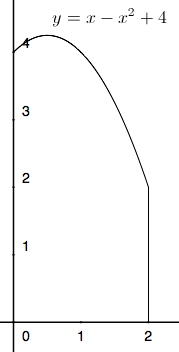#### Question 3 3. The average value for this continuous function over the shaded region is 2. According to the average value theorem, how many times does this function pass through the value 2?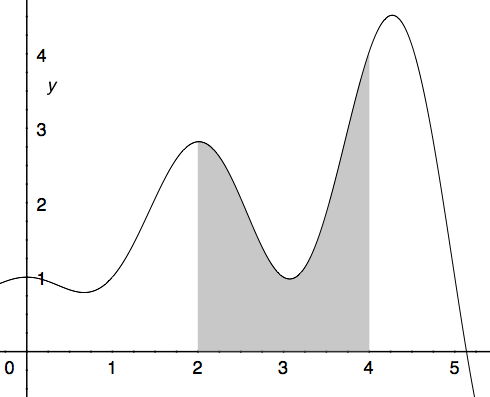#### Question 4 4. Which integral below is equal to the following integral?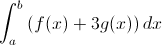#### Question 5 5. Evaluate the following.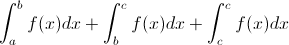### Page 2

#### Question 6 6. The following integral can be re-written as: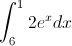#### Question 7 7. Given the following information, what does z equal?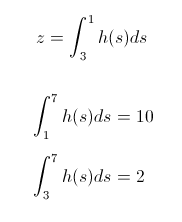#### Question 8 8. Given a continuous function f(x) that satisfies the equation below, which of the following is true, according to the average value theorem?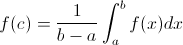#### Question 10 10. What is the area under the curve below using a single trapezoid?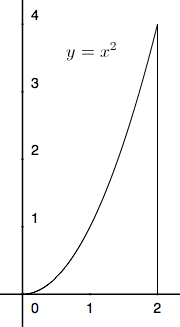### Page 3

#### Question 11 11. What is the two-term left-side Riemann sum for y between 0 and 2?#### Question 12 12. Use three trapezoids to find the area under the curve shown below between x=0 and x=3.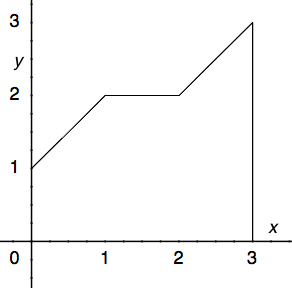#### Question 13 13. What is the one-term left-side Riemann sum for y=f(x) from 0 to 2 for the graph below?#### Question 14 14. Which of the following represents a four-term right-handed Riemann sum for y=f(x)?#### Question 15 15. Use the average value theorem to determine the average value of f(x) between 0 and 2.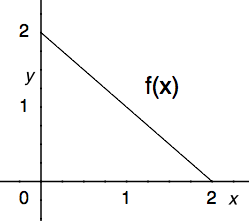### Page 4

#### Question 16 16. Which of the following is NOT either a left, right, or center Riemann sum for f(x) between 0 and 4?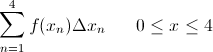#### Question 17 17. What is the area under the curve below using two trapezoids?#### Question 18 18. Which integral below is equal to the following integral?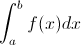#### Question 20 20. What is the area under the curve below using a single trapezoid?#### Approximating Definite Integrals Chapter Exam Instructions

Choose your answers to the questions and click 'Next' to see the next set of questions. You can skip questions if you would like and come back to them later with the yellow "Go To First Skipped Question" button. When you have completed the practice exam, a green submit button will appear. Click it to see your results. Good luck!

Support